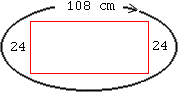Date: Sat, 14 Aug 1999 12:53:41 -0600 (CST)
Name: mel
Who is asking: Student
Level: Middle

Question:
The length of a rectangle is IS 3 1/2 times its width. Its perimeter is 108cm. Find its length and width. Choices for the width: 12,18,24

Hi Mel
 Since you are given three choices for the width you can do this problem by trying the three possibilities to see what works.    If the width is 24 cm then, since the perimeter is 108 cm, the two other sides have a total length of 108 - 2(24) = 60 cm. Hence the length of the rectangle is 60/2 = 30 cm. But 30/24 = 1 1/4, so the length is 1 1/4 times its width not 3 1/2 times its width.    Now try a width of 18 cm and 12 cm and see which works. Cheers PennyGo to Math Central

To return to the previous page use your browser's back button.